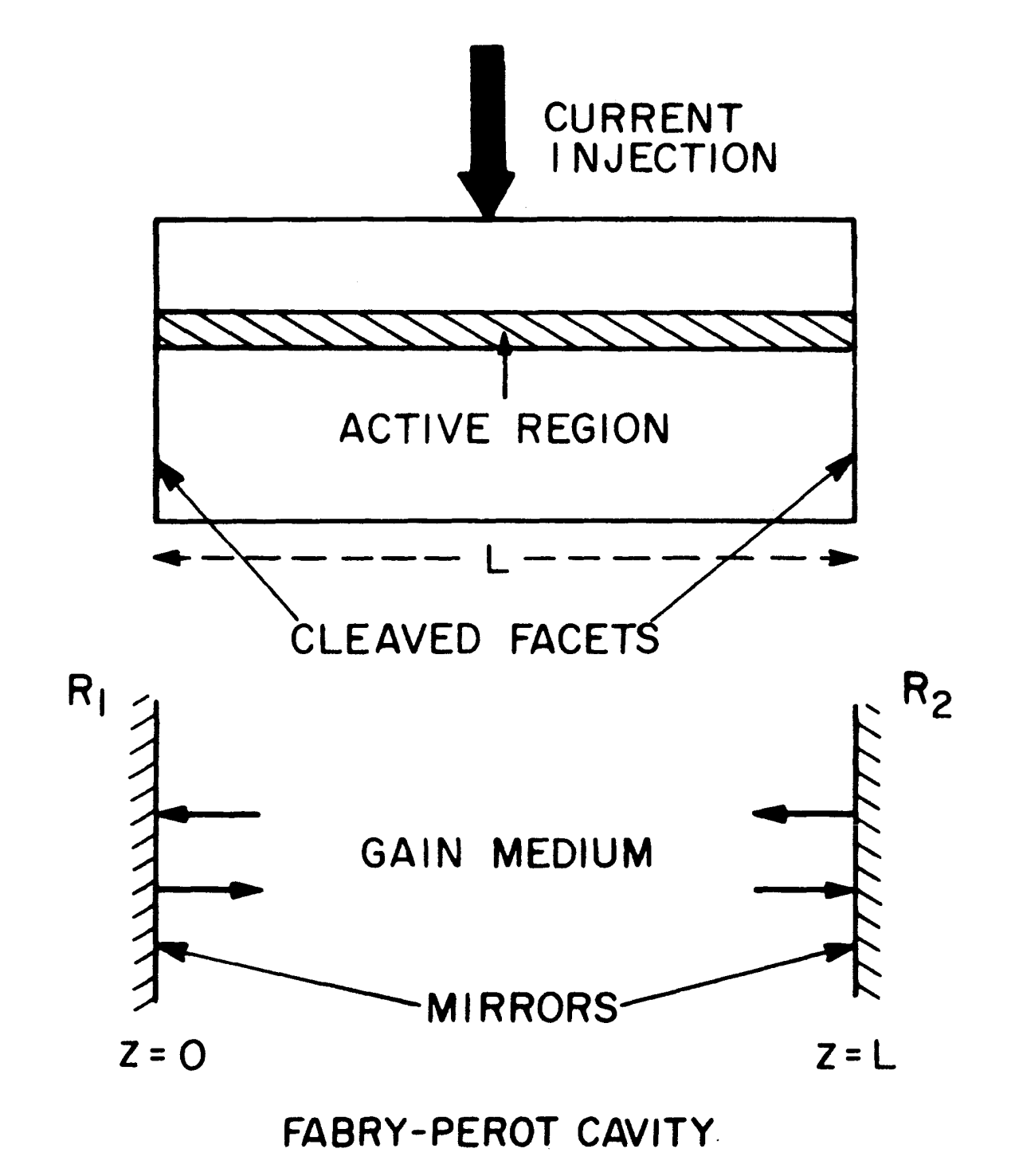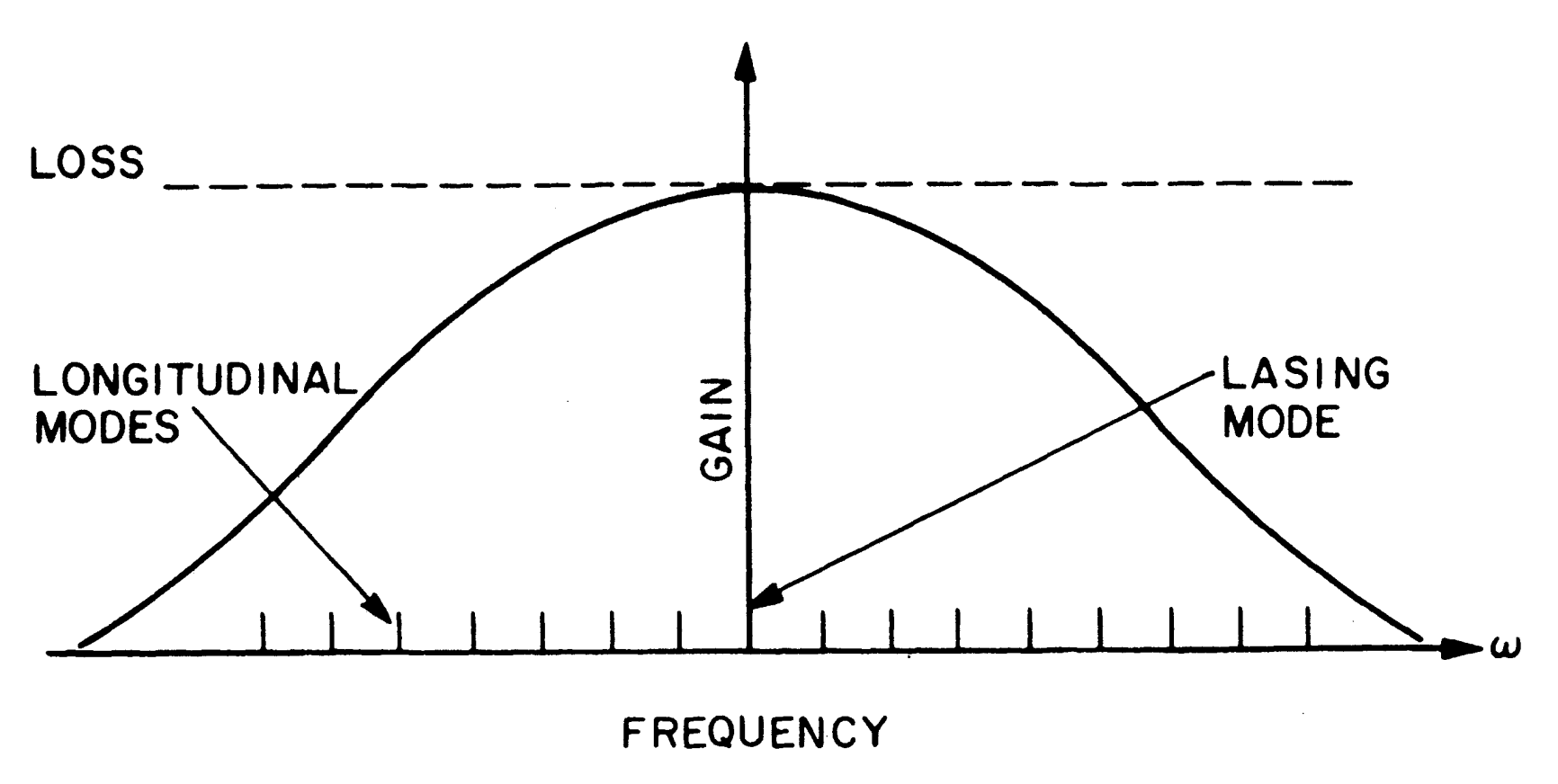# Threshold Condition and Longitudinal Modes of Semiconductor Lasers

This is a continuation from the previous tutorial - Maxwell's equations for semiconductor lasers.

The plane-wave solution, Equation (2-2-21) [refer to the Maxwell's equations for semiconductor lasers tutorial], of the wave equation obtained in the Maxwell's equations for semiconductor lasers tutorial can be used to obtain an estimate of the laser frequency and the optical gain required for the onset of oscillations.

It should be kept in mind that the lasing modes are never plane waves; another later tutorial considers the spatial variations of the lasing modes.

Nonetheless, the threshold condition derived here is reasonably accurate and is helpful in understanding the essential physics of the lasing process.

Consider a semiconductor laser of length $$L$$ shown schematically in Figure 2-1 below.Figure 2-1. Schematic illustration of a semiconductor laser and its associated Fabry-Perot (FP) cavity. The cleaved facets act as partially reflecting mirrors.

The thin central region provides the optical gain. The cleaved facets form a Fabry-Perot (FP) cavity and provide the feedback for the onset of laser oscillations.

If the $$z$$ axis is taken along the cavity length, the optical field in the plane-wave approximation is given by Equation (2-2-21) [refer to the Maxwell's equations for semiconductor lasers tutorial], i.e.,

$\tag{2-3-1}\mathbf{E}=\hat{\mathbf{x}}E_0\exp(\text{i}\hat{\beta}z)$

where from Equations (2-2-22) and (2-2-23) [refer to the Maxwell's equations for semiconductor lasers tutorial] the complex propagation constant takes the form

$\tag{2-3-2}\hat{\beta}=\mu{k_0}+\text{i}\alpha/2$

Equation (2-2-24) [refer to the Maxwell's equations for semiconductor lasers tutorial]  for the refractive index shows that $$\mu$$ changes with external pumping of the semiconductor laser, as also observed experimentally.

The physical reason for this change is related to the high density of charge carriers inside the active region. Several mechanisms contribute to $$\text{Re}(\boldsymbol{\chi}_\text{p})$$. The most dominant contribution is due to the band-gap shrinkage occurring in the presence of charge carriers.

Generally, however, $$|\text{Re}(\boldsymbol{\chi}_\text{p})|\ll\epsilon_\text{b}$$, and Equation (2-2-24) [refer to the Maxwell's equations for semiconductor lasers tutorial] can be approximated by

$\tag{2-3-3a}\mu\approx\mu_\text{b}+\Delta\mu_\text{p}$

where $$\mu_\text{b}=\sqrt{\epsilon_\text{b}}$$ is the background refractive index of the unpumped material and $$\Delta\mu_\text{p}$$, the amount by which it changes in the presence of charge carriers, is given by

$\tag{2-3-3b}\Delta\mu_\text{p}\approx\text{Re}(\boldsymbol{\chi}_\text{p})/2\mu_\text{b}$

Usually $$\text{Re}(\boldsymbol{\chi}_\text{p})$$ is negative, making $$\Delta\mu_\text{p}$$ also negative.

Even though the reduction in the refractive index is often less than 1%, it significantly affects the static, dynamic, and spectral characteristics of a semiconductor laser. This is in contrast to other kinds of lasers, such as a gas laser, where $$\Delta\mu_\text{p}\approx0$$.

Next consider the absorption coefficient given by Equation (2-2-25) [refer to the Maxwell's equations for semiconductor lasers tutorial].

It has three contributions arising from different sources.

The term $$\text{Im}(\boldsymbol{\chi}_0)$$ accounts for the material absorption, while $$\text{Im}(\boldsymbol{\chi}_p)$$ is responsible for its reduction with the external pumping.

It is often convenient to describe their combined effect as the net gain $$g$$, defined as

$\tag{2-3-4}g=-\frac{k_0}{\mu_\text{b}}\text{Im}(\boldsymbol{\chi}_0+\boldsymbol{\chi}_\text{p})$

The last term in Equation (2-2-25) accounts for other internal losses that generally occur in a semiconductor laser.

Several mechanisms such as free-carrier absorption and scattering at the heterostructure interfaces may contribute to the internal loss. Since the individual contribution of these and other internal losses is often difficult to estimate, they are collectively accounted for through $$\alpha_\text{int}=k_0\sigma/(\epsilon_0\omega\mu)$$.

The net absorption coefficient then becomes

$\tag{2-3-5}\alpha=-\Gamma{g}+\alpha_\text{int}$

The constant factor $$\Gamma$$ is introduced here phenomenologically and and its use will be justified in later tutorials.

Physically, it accounts for the reduction in gain that occurs because of the spreading of the optical mode beyond the active region. It is known as the confinement factor or the filling factor and represents the fraction of the mode energy contained in the active region.

To obtain the threshold condition, we require that the optical field Equation (2-3-1) should reproduce itself after each round trip under steady-state or continuous-wave (CW) operating conditions.

If $$R_1$$ and $$R_2$$ are the facet reflectivities at two ends, the net change in the amplitude after one round trip is set to unity at the laser threshold. This leads to the condition

$\tag{2-3-6}(R_1R_2)^{1/2}\exp(2\text{i}\hat{\beta}L)=1$

Equating the real and imaginary parts of Equation (2-3-6) and substituting $$\hat{\beta}$$ from Equation (2-3-2), we obtain

$\tag{2-3-7}(R_1R_2)^{1/2}\exp(-\alpha{L})=1$

$\tag{2-3-8}\sin(2\mu{k_0}L)=0$

The first condition (2-3-7) gives the threshold gain. Using (2-3-5), it can be written in the form

$\tag{2-3-9}\Gamma{g}=\alpha_\text{m}+\alpha_\text{int}$

where

$\tag{2-3-10}\alpha_\text{m}=\frac{1}{2L}\ln\left(\frac{1}{R_1R_2}\right)$

is the mirror loss and accounts for the radiation escaping from the FP cavity because of finite facet reflectivities.

Equation (2-3-9) expresses the fact that the gain due to external pumping must balance the total losses.

We remark that this is only approximately true since the effect of spontaneous emission has been ignored in this simple analysis. The contribution of spontaneous emission, invariably present in any laser, is to slightly lower the gain required to reach threshold from that given by Equation (2-3-9). The effects of spontaneous emission are discussed in a later tutorial.

The condition (2-3-8) can be used to obtain the lasing frequency. However, because of the periodic nature of trigonometric functions, Equation (2-3-8) has multiple solutions

$\tag{2-3-11}2\mu{k_0}L=2m\pi$

where $$m$$ is an integer.

Using $$k_0=2\pi\nu/c$$, the lasing frequency $$\nu$$ is given by

$\tag{2-3-12}\nu=\nu_m=mc/(2\mu{L})$

where $$\nu_m$$, often referred to as the cavity-resonance frequency, is the frequency of the $$m$$th longitudinal mode of an FP cavity of the optical length $$\mu{L}$$.

Equation (2-3-12) shows that the laser tends to oscillate at a frequency that coincides with that of a longitudinal mode supported by the FP cavity.

Which one and how many of them reach threshold depends on details of the gain spectrum such as the gain bandwidth and the gain-broadening mechanism (whether homogeneous or inhomogeneous).

In the case of homogeneous broadening only one longitudinal mode, whose frequency nearly coincides with the gain-peak frequency, reaches threshold, and the laser maintains the single-longitudinal-mode operation even in the above-threshold regime (see Figure 2-2) .Figure 2-2. Schematic illustration of the gain profile and the longitudinal modes of a semiconductor laser. For the lasing mode in the vicinity of the gain peak, the threshold is reached when gain equals loss.

The longitudinal-mode spacing can be obtained from Equation (2-3-12). However, it is important to remember that in a semiconductor laser the refractive index $$\mu$$ varies with the frequency $$\nu$$.

Using the relation

$\tag{2-3-13}\Delta(\mu\nu)=\mu(\Delta\nu)+\nu(\Delta\mu)$

where $$\Delta$$ denotes a small change, the intermode spacing is given by

$\tag{2-3-14}\Delta\nu=c/(2\mu_gL)$

where

$\tag{2-3-15}\mu_g=\mu+\nu(\partial{\mu}/\partial\nu)$

is the group index of the dispersive semiconductor material.

A feature unique to semiconductor lasers is that the longitudinal-mode frequencies and their separation vary with the external pumping because of the refractive-index variations as indicated by Equation (2-3-3a).

For the purpose of a rough estimate, consider an InGaAsP semiconductor laser in which cavity length $$L=250$$ μm. For this material in the wavelength range of 1.3-1.6 μm, $$\mu\approx3.5$$ and $$\mu_g\approx4$$. The longitudinal mode spacing from Equation (2-3-14) is estimated to be $$\Delta\nu\approx150$$ GHz or $$\Delta\lambda=\lambda^2\Delta\nu/c\approx1$$ nm. If we use the cleaved-facet reflectivity ($$R_1=R_2\approx0.32$$) the facet loss $$\alpha_\text{m}$$ from Equation (2-3-10) is $$\sim45\text{ cm}^{-1}$$. Using typical values for $$\Gamma$$ and $$\alpha_\text{int}$$ of 0.5 and $$\sim30\text{ cm}^{-1}$$ respectively in Equation (2-3-9), a material gain $$g$$ of $$150\text{ cm}^{-1}$$ is required to achieve threshold.

From the viewpoint of device operation, the quantity of practical interest is not the threshold gain itself but the threshold current density $$J_\text{th}$$ required to achieve the gain.

To obtain a relation between the gain $$g$$ and the injected current density $$J$$, it becomes essential to consider the response of the semiconductor material to the optical field.

This is considered in the next tutorial.

The next tutorial covers the topic of gain and stimulated emissions of semiconductor lasers.